Courses

# Levelling and Contouring Civil Engineering (CE) Notes | EduRev

## Civil Engineering (CE) : Levelling and Contouring Civil Engineering (CE) Notes | EduRev

The document Levelling and Contouring Civil Engineering (CE) Notes | EduRev is a part of the Civil Engineering (CE) Course Topic wise GATE Past Year Papers for Civil Engineering.
All you need of Civil Engineering (CE) at this link: Civil Engineering (CE)

Question 1: A series of perpendicular offsets taken from a curved boundary wall to a straight survey line at an interval of 6 m are 1.22,1.67, 2.04, 2.34, 2.14, 1.87, and 1.15 m. The area (in m2, round off to 2 decimal places) bounded by the survey line, curved boundary wall, the first and the last offsets, determined using Simpson's rule, is__________.    [2019 : 2 Marks, Set-II]
Solution: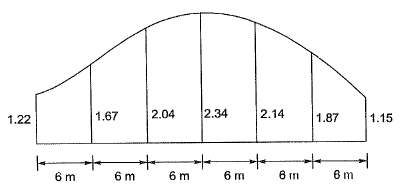Area by Simpson’s rule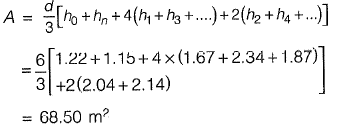Question 2: A staff is placed on a benchmark (BM) of reduced level (RL) 100.000 m and a theodolite is placed at a horizontal distance of 50 m from the BM to measure the vertical angles. The measured vertical angles from the horizontal at the staff readings of 0.400 m and 2.400 m are found to be the same. Taking the height of the instrument as 1.400 m, the RL (in m) of the theodolite station is_______.    [2019 : 2 Marks, Set-I]
Solution: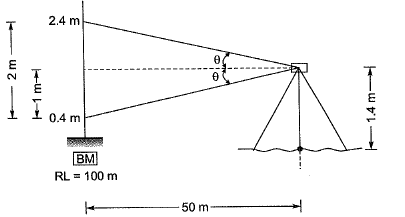HI = RL of BM + BS
= 100 + (0.4 + 1)
= 101.4
RL of Theodolite station
= Hl-height
= 101.4 - 1.4
= 100 m

Question 3: A level instrument at a height of 1.320 m has been placed at a station having a Reduced Level (RL) of 112.565 m. The instrument reads -2.835 m on a levelling staff held at the bottom of a bridge deck. The RL (in m) of the bottom of the bridge deck is    [2018 : 2 Marks, Set-II]
(a) 116.720
(b) 116.080
(c) 114.080
(d) 111.050
Answer: (a)
Solution: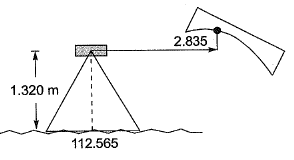RL of bottom of bridge deck
= 112.565 + 1.320 + (2.835)
= 116.720 m

Question 4: An observer standing on the deck of a ship just sees the top of a lighthouse. The top of the lighthouse is 40 m above the sea level and the height of the observer’s eye is 5 m above the sea level. The distance (in km, up to one decimal place) of the observer from the lighthouse is _____. [2017 : 2 Marks, Set-II]
Solution: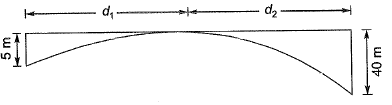Distance of observer from the lighthouse,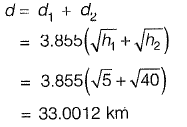Question 5: The vertical angles subtended by the top of a tower T at two instrument stations set up at P and Q, are shown in the figure. The two stations are in line with the tower and spaced at a distance of 60 m. Readings taken from these two stations on a leveling staff placed at the benchmark (BM = 450.000 m) are also shown in the figure. The reduced level of the top of the tower T (expressed in m) is__________    [2016 : 2 Marks, Set-I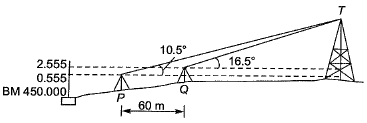Solution: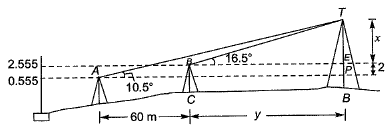In ΔBET,
tan 16.5° = x/y
⇒ y = 3.3759x  .....(i)
In ΔAOT,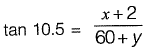......(ii)
Put value of y in eg. (ii)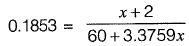11.1203 + 0.6255x = x + 2
x = 24.3568 m
So, the reduced level of tower,
T = 450.000 + 2.555 + 24.356

= 476.911 m

Question 6: The staff reading taken on a workshop floor using a level is 0.645 m. The inverted staff reading taken to the bottom of a beam is 2.960 m. The reduced level of the floor is 40.500 m. The reduced level (expressed in m) of the bottom of the beam is    [2016 : 1 Mark, Set-I]
(a) 44.105
(b) 43.460
(c) 42.815
(d) 41.145
Answer: (a)
Solution: Height of instrument, HI
= R.L. of floor + staff reading from floor
= 40.500 + 0.645
= 41.145 m
R.L. of bottom of beam
= HI + inverted staff reading taken from bottom of beam
= 41.145 + 2.960
= 44.105 m

Question 7: Two pegs A and B were fixed on opposite banks of a 50 m wide river. The level was set up at A and the staff readings on Pegs A and B were observed as 1.350 m and 1.550 m, respectively. Thereafter the instrument was shifted and set up at B. The staff readings on Pegs B and A were observed as 0.750 m and 0.550 m, respectively. If the R.L. of Peg A is 100.200 m, the R.L. (in m) of Peg B is _____.    [2015 : 2 Marks, Set-II]
Solution: Staff readings shows that station B is below station A.
a1 = 1.350
a2 = 0.550
b3 = 1.550
b2 = 0.750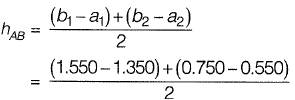= 0.200
RL of B = RL of A - 0.200
= 100.200-0.200
= 100.000 m

Question 8: The combined correction due to curvature and refraction (in m) for distance of 1 km on the surface of Earth is    [2015 : 1 Mark, Set-II]
(a) 0.0673
(b) 0.673
(c) 7.63
(d) 0.763
Answer: (a)
Solution: Correction due to curvature,
Cc = -0.0785 d2
Correction due to refraction,
Cr = +0.0112 d2
Composite correction,
C = -0.0785d2 + 0.0112 d2
= -0.0673 d2
where d is in km, C is in m,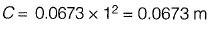Question 9: In a leveling work, sum of the Back Sight (B.S.) and Fore Sight (F.S.) have boon found to be 3.085 m and 5.645 m respectively. If the Reduced Level (R.l .) of the starting station is 100.000 m, the R.L. (in m) of the last station is    [2015 : 1 Mark, Set-II]
Solution: Using Rise and Fall method,
∑F.S. > ∑B.S.
∴ Fall = ∑F.S. - ∑B.S. = R.L. of first station - R.L. of last station = 5.645-3.085 = 2.56 m
R.L. (last station) = R.L. (first station) - Fall
= 100 - 2.56 = 97.44 m

Question 10: Which of the following statements is FALSE?    [2015 : 1 Mark, Set-I]
(a) Plumb line is along the direction of gravity
(b) Mean Sea Level (MSL) is used as a reference surface for establishing the horizontal control
(c) Mean Sea Level (MSL) is a simplification of the Geoid
(d) Geoid is an equi-potential surface of gravity
Answer: (b)
Solution: Mean sea level (MSL) is used as a reference surface for establishing the vertical control.

Question 11: A levelling is carried out to establish the Reduced Levels (RL) of point R with respect to the Bench Mark (BM) at P. The staff readings taken are given below:    [2014 : 2 Marks, Set-I]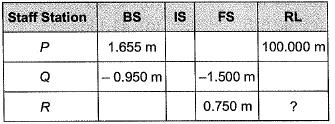If RL of P is +100.000 m, then RL (in m) of R is
(a) 103.355
(b) 103.155
(c) 101.455
(d) 100.355
Answer: (c)
Solution:
HI = RL + BS
and RL = HI - FS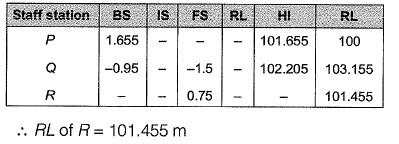Question 12: The Reduced Levels (RLs) of the points Pand Q are +49.600 m and +51.870 m respectively. Distance PQ is 20 m. The distance (in m from P) at which the +51.000 m contour cuts the line PQ is    [2014 : 1 Mark, Set-I]
(a) 15.00
(b) 12.33
(c) 3.52
(d) 2.27
Answer: (b)
Solution: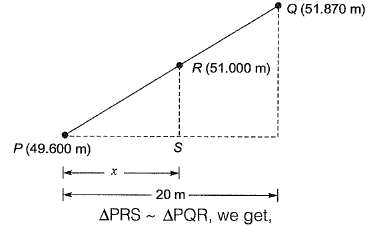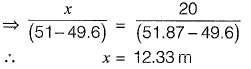Question 13: The horizontal distance between two stations P and Q is 100 m. The vertical angles from P and Q to the top of a vertical tower at T are 3° and 5° above horizontal, respectively. The vertical angles from P and Q to the base of the tower are 0.1 ° and 0.5° below horizontal, respectively. Stations P, Q and the tower are in the same vertical plane with P and Q being on the same side of T. Neglecting earth's curvature and atmospheric refraction, the height (in m) of the tower is    [2012 : 2 Marks]
(a) 6.972
(b) 12.387
(c) 12.540
(d) 128.745
Answer: (b)
Solution: x (tan 3 + tan 0.1) = (x - 100) (tan5° + tan0.5°)
⇒ x = 228.758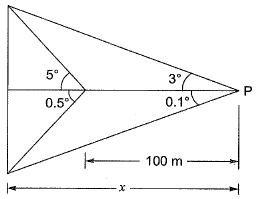Height of tower= 228.758 (tan 3° + tan0.1°)
= 12.387 m
Note: Question has erroreous data, hence many other answers are also possible.

Question 14: Which of the following errors can be eliminated by reciprocal measurements in differential leveling?    [2012 : 1 Mark]
I. Error due to earth’s curvature
II. Error due to atmospheric refraction
(a) Both I and II
(b) I only
(c) II only
(d) Neither I nor II

Answer: (a)
Solution:
Reciprocal levelling eliminates the errors due to
(i) Error in instrument adjustment.
(ii) Combined effect of earth’s curvature and the refraction of the atmosphere.
(iii) Variation in the average refraction.

Question 15: Curvature correction to a staff reading in a differential leveling survey is    [2011 : 1 Mark]
(a) always subtractive
(b) always zero
(c) always additive
(d) dependent on latitude
Answer: (a)

Question 16: A bench mark has been established at the soffit of an ornamental arch at the known elevation of 100.0 m above mean sea level. The back sight used to establish height of instrument is an inverted staff reading of 2.105 m. A forward sight reading with normally held staff of 1.105 m is taken on a recently constructed plinth. The elevation of the plinth is    [2010 : 2 Marks]
(a) 103.210 m
(b) 101.000 m
(c) 99.000 m
(d) 96.790 m
Answer: (d)
Solution: Height of instrument = BM + Back sight
Since the staff is inverted, the back sight will be negative.
∴ Height of instrument
= 100 + (- 2.105) = 97.897 m
Elevation of plinth = Height of instrument- Fore sight
= 97.895-1.105 = 96.790 m

Offer running on EduRev: Apply code STAYHOME200 to get INR 200 off on our premium plan EduRev Infinity!

69 docs

,

,

,

,

,

,

,

,

,

,

,

,

,

,

,

,

,

,

,

,

,

;